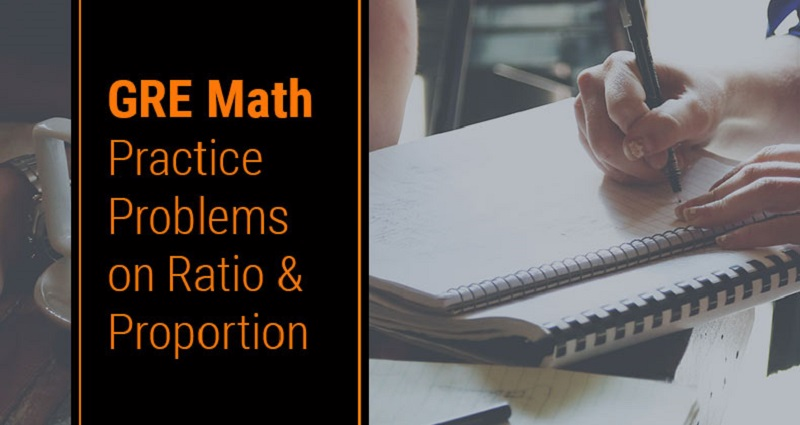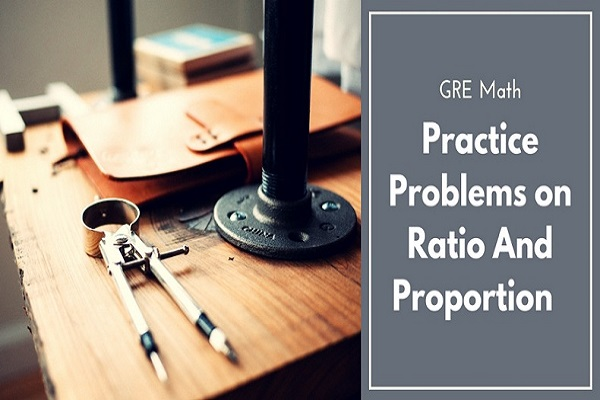X

# GRE Math: Practice Problems on Ratio And Proportion

Jun 20, 2018Ratio and proportion are one of those concepts that may pop up in about any GRE numerical problem. You may have to calculate the ratio between 2 angles forming intersecting lines, or you may have to calculate the ratio of speeds a person drove at.

With such broad applications, it’s imperative for you to know about these concepts in minute details. Here, in this post, we have come up with a few sample problems for your practice. If you are a serious GRE aspirant, consider going through them all without any further ado.Q1. A bag contains 50 P, 25 P and 10 P coins in the ratio 5: 9: 4, amounting to Rs. 206. Find the number of coins of each type respectively.

A) 360, 160, 200

B) 160, 360, 200

C) 200, 360,160

D) 200,160,300

Solution:

Let the no. of coins be 5x ,9x , 4x respectively

Now given total amount = Rs.206

Therefore, (.50)(5x) + (.25)(9x) + (.10)(4x) = 206

Solving the equation, we get x = 40

=> No. of 50p coins = 200

=> No. of 25p coins = 360

=> No. of 10p coins = 160

Q2. A mixture contains alcohol and water in the ratio 4 : 3. If 5 liters of water is added to the mixture, the ratio becomes 4: 5. Find the quantity of alcohol in the given mixture.

A) 10

B) 12

C) 15

D) 18

Solution:

Let the quantity of alcohol and water be 4x litres and 3x litres respectively

4x/(3x+5) = 4/5

or, 20x = 4(3x+5)

or, 8x = 20

or, x = 2.5

Quantity of alcohol = (4 x 2.5) litres = 10 litres.

Q3. If Rs. 782 be divided into three parts, proportional to 12:23:34, then the first part is?

A) Rs. 182

B) Rs. 190

C) Rs. 192

D) Rs. 204

Solution:

Rs.(782*6/23)

Given ratio = 1/2 : 2/3 : 3/4 = 6 : 8 : 9

1st part = Rs.(782* 6/23) = Rs. 204

Q4. Seats for Mathematics, Physics and Biology in a school are in the ratio 5 : 7 : 8. There is a proposal to increase these seats by 40%, 50% and 75% respectively. What will be the ratio of increased seats?

A) 2 : 3 : 4

B) 6 : 7 : 8

C) 6 : 8 : 9

D) None of these

Answer: A) 2 : 3 : 4

Solution:

Originally, let the number of seats for Mathematics, Physics and Biology be 5x, 7x and 8x respectively.

Number of increased seats are (140% of 5x), (150% of 7x) and (175% of 8x).

or, (140/100 x 5x) , (150/100 x 7x) and (175/100 x 8x)

or, 7x, 21x/2 and 14x

Therefore, the required ratio = 7x : 21x/2 : 14x

or, 14x : 21x : 28x

or, 2 : 3 : 4

Q5. In a mixture 60 litres, the ratio of milk and water 2 : 1. If this ratio is to be 1 : 2, then the quanity of water to be further added is:

A) 20 litres

B) 30 litres

C) 40 litres

D) 60 litres

Solution:

Quantity of milk = (60 x 2/3) litres = 40 litres.

Quantity of water in it = (60 - 40) litres = 20 litres.

New ratio = 1 : 2

Let quantity of water to be added further be x litres.

Then, milk : water = (40/20+x).

Now, (40/20+x) = 1/2

or, 20 + x = 80

or, x = 60

Therefore, quantity of water to be added = 60 litres.

Q6. The ratio of Alice's pay to Bob's pay is 5:4. The ratio of Bob's pay to Charlie's pay is 10:9. If Alice is paid $75, how much is Charlie paid? A)$55

B) $57 C)$54

D) $40 Answer: C)$54

Solution:

Since the ratio of Alice's pay to Bob's pay is , Bob's pay must be b, where 5/4 = 75/b.

Cross-multiplying the denominators, we get , 5b = 4 (75); so b = 60.

Continuing in the same way, we compare Bob to Charlie:

10/9 = 60/c

or, 10c = 9 (60)

or, c = 54

Thus, Charlie is paid \$54.

All the best for your GRE examination!

Article Posted in: Exam Preparation### MyPrivateTutor UAE

MyPrivateTutor is an online marketplace for all kinds of tutoring and learning services. We provide a platform to help learners find home tutors, online tutors, expert trainers,tutorial centers, training institutes and online tutoring schools. Learners can also find local classes, workshops, online courses and tutorials on a huge variety of topics. We operate in 12 countries worldwide and our community of learners, teachers and training businesses numbers over 200,000. We provide an opportunity for individual tutors and tutoring businesses to promote and grow their business.